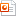검색어 입력폼

# MATLAB] Heat_exchange.

저작시기 2009.06 |등록일 2009.06.17MS 파워포인트 (ppt) | 26페이지 | 가격 2,500원

## 소개글

Improve euler’s method와 Runge-Kutta method를 이용한 문제 분석

## 목차

Ⅰ . Introduce problem 1

Ⅱ . Solve by Improved Euler’s Method and Runge-Kutta Method

Ⅲ . Graph and Table

Ⅳ . Introduce problem 2.

Ⅴ. Solve by Improved Euler’s Method and Runge-Kutta Method

Ⅵ. Graph and Table

## 본문내용

Newton’s law of coolling
Newton’s law of cooling states that the rate of change in the temperature T(t) of a body is proportional to the difference between the temperature of the medium M(t) and the temperature of the body. That is
where K is a constant. Let K=1 and the temperature of the medium be constant, M(t)=70℉. If the body is initially at 100℉, use the improved Euler’s method and fourth order Runge-Kutta method to approximate the temperature of the body after
(1) 1 minute, (2) 2minute.
Problem 1.
`
Newton’s law of coolling
Solve the differential equation (Analytic method)
Analytic solution
Problem 1.
`
Improved Euler’s method
function y=improve(h)
x=0;
y=100;
xa=[0:h:10];
ya=70+30./exp(xa)
plot(xa,ya,`k`),hold on % Exact Sol
a=1;
while a<=1/h
f1=70-y;
yold=y; % Improved
x=x+h; % Euler`s
y=yold+h*f1; % Method

없음